﻿ The Electrical Vortex Non-Solenoidal Fields
 Sergey B. Alemanov  http://alemanow.narod.ru  alemanow@mail.ru Electrical Vortex Non-Solenoidal Fields (Vortex and solenoidal fields are different concepts)

Following the analysis of the electrical and magnetic fluxes properties in electrodynamics the errors have been found. It has been discovered that not all postulates comply with the experimental facts, and vortex electric flows can have open induction lines. For example, in the vortex electric flows only the streamlines of the electric displacement are always closed, and the electric displacement lines may be open (electric displacement current is measured in amperes, and electric displacement flow in coulombs). Below we shall review the electrodynamics of the moving bodies on the example of the magnets and charges movement.

When the magnet is moving, then the current of magnetic induction is moving together with it. Knowing the motion speed v and the value of magnetic induction B, it is possible, according to the electrodynamics formula of fields transformation D = ε0[vB], to calculate the displacement D of the emerging eddy electric fields. Herewith the appearing electric induction is always transverse to the direction of motion. It is possible to formulate the rule of origin for electric induction under the condition of rectilinear motion: if to dispose the right hand palm so four fingers shows the motion direction of the magnetic flow (the field), connected with moving magnet, and the vector B fells into palm, then the moved aside big finger will indicate the direction of vector D. The given rule is like the rule for Lorenz' force, but on the contrary (the difference is in frame). In the first case the charge moves, but the magnet rests. Here the magnet moves, but the charge, which points the direction for lines of force of electric induction, is immovable. So, there it is the rule for left hand, but here, on the contrary, it is the rule for right hand. Thereby, if the charge moves, but the magnet is immovable, then the rule of left hand uses for determination of the force. But if the magnet moves, but the charge rests, then the rule of right hand uses for determination of the force.

The origin of electric force is connected with that, the vortex electric field D = ε0[vB] appears around moving magnet (the magnetic field does not act on immovable charges).

«The magnetic field acts only on moving electrically charged particles and bodies, ...»
B.M. Yavorskiy, A.A. Detlaf. Handbook of physics. Moscow: "Science". 1996 p.232. (In Russian)

In common literature on electrodynamics there is no any difference between electric vortex field and solenoidal field, but these are different notions. The sign of solenoidal field is the closed lines of electric induction (the flow of vector D through the closed surface is a zero), but for the vortex field the sign is following: the work of forces can be different from zero under the condition of motion along a closed line. That is to say, the vortex fields can agitate the rotational currents.

«The work of forces of vortex electric field can be different from zero, when the electric charge is moving along a closed line.»
Physics. O.F.Kabardin. 1991. P.189. (In Russian)

Unlike to the vortex electric flows the work of force of the potential field at the electric charge motion along the closed line is always equal to zero. It should be highlighted that when speaking of motion along the closed line, it is not necessarily the motion along the induction (power) field lines. Although the work of force vortex electric flow at the motion along the closed line can be different from zero, the lines of the field force can be either closed or open. For example, at the magnet motion the vortex electric flow emerges, but depending on the orientation of magnet the field may be either a solenoidal or not.

Let's take such example: the magnet moves evenly, rectilinearly, and it's poles are oriented transversely to direction of motion. According to the rule of origin for electric induction (D = ε0[vB] that is the rule of right hand), the appearing vortex electric flow is not a solenoidal, since the lines of electric induction are not closed. Its begins in one conditional area of disturbance
(+), accompanies the moving magnet, and it finish in another area of disturbance (-). For presentation it is enough to consider only two areas (+) and (-), represented on figure. These dissimilar areas of disturbance appears because that flow of magnetic induction inside the magnet has the inverse direction, that outside the magnet. In the magnet there is no polarization, since Lorentz force acts on the moving charges, balancing the eddy electric field. It should also be noted that, although at such magnet motion the eddy electric field is not closed, but the associated electrical displacement current (currents are always closed). The lines of the electric displacement field (electric induction lines) shall not be confused with the electric displacement field current lines (displacement current lines). In given example, for clarity, it is possible to present a intensity of electric field through the Lorenz' force, if to take the frame, in which the magnet rests, and the test charge moves.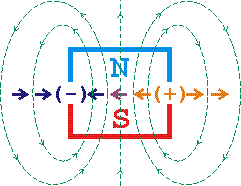On the Fig.1 the moving magnet is conditionally represented (motion is toward to the text, magnet is moving away). N and S are poles of magnet. The direction of lines of electric induction, appearing when the magnet is moving, specified by arrows "->" and "<-". Part of the lines begins in positive area (+) and finishes in negative area (-), the areas are placed on the ends of magnet. The flow of electric induction through closed surface is not a zero; that is to say, these areas of disturbance are moving electric charges.

«The flow of vector D through any closed surface is equal to algebraic amount of external charges, covered by this surface. : In the electrodynamics these postulates has the same role, as Newton' laws in classical mechanics ...»
Electromagnetism. I.E.Irodov. 2000. P.288. (In Russian)

Thereby, according to postulate, it is necessary to consider the appearing dissimilar areas of disturbance (+) and (-) to electric charges, or it is necessary to change the postulate, representing the Maxwell equation. It is interesting, that a part of lines of electric induction, which placed frontal and behind magnet, starts and finish at infinity, since the distribution of magnetic induction around magnet has not determined borders. (In this example, for illustrative purposes only, a magnet can be represented as the current ring.)

Now we shall consider the motion of two transversely oriented opposite electric charges.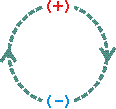Direction of movement  --->

The figure shows two moving opposite charges (+) and (-). The arrows indicate the direction of the emerging current of the electric displacement. Knowing that in the middle between the opposite charges D = q/r2, it is possible, according to the electrodynamic fields conversion formula B = μ0[vD], to find the emerging magnetic induction B = μ0qv/r2, where q - charges, r - distance from the center, v - motion speed, μ0 - magnetic constant.

The considered transversely oriented motion of the electric charges and magnet is somewhat symmetrical. That means, that when charges move, magnetic field emerge. If vice versa a magnet moves, the reverse process appear and induced charges emerge.

Let's consider another example: magnet moves rectilinearly, but its poles are oriented longitudinally to direction of motion. According to the rule for origin of electric induction (D = ε0[vB] is the rule of right hand), the appearing rotational electric flow is solenoidal, since in this case the inductive lines become closed lines. Usually in books on the electrodynamics such moving magnet is considered, and the wrong conclusion is thereof done, that vortex electric field is always solenoidal, herewith it is forgotten, that poles of the magnet can be oriented not only along the direction of motion, but across also.

«The vortex electric field differs from electrostatic field that it is not related with any electric charges and its lines of intensity are closed lines.»
Physics. O.F.Kabardin. 1991. С.189. (In Russian)

But at the transverse motion of the magnet the force lines of the vortex electric field can be open and, accordingly, the induction flow through the closed surface is not equal to zero. That means that in contemporary electrodynamics there are inconsistent facts. Surprisingly, bur during the history of magnetism study the transverse motion of the magnet has not been considered, leading to a revision of the principles of electrodynamics, that is the revision of the postulates, that in the electrodynamics play the same role as Newton's laws in the classical mechanics. It turns out, that not only particles can have charges, but the field perturbed region (without particles) may also have charges, where the electrical induction flow through the closed surface is not equal to zero. Thus, vortex electric fields may have not only the form of closed induction flows, but also the form of induced electrical charges, on which the laws inherent to the electric charges are distributed. For example, charge conservation law, which means, that if somewhere a perturbed region with positive sign emerges, the negative regions emerges too for sure.

It is wrong to assume that the vortex electric field is generated only by a variable magnetic field. The variable electric field (nonstatic electric field) also generates a vortex electric field. Let's consider an example: if a frame placed between the plates of a capacitor, and stabilize the current in the condenser, making it a constant, there will be no circular current in the frame at any position, despite the fact that there will be the constant displacement current between the capacitor plates at that time (constant magnetic field). This means the vortex current will not emerge in the frame, since the magnetic field does not change, but between the plates of the condenser the force work of the electric field at the motion of the electric charge along the closed line can be different from zero, and this is a sign of the vortex electric field. When a charge moves in one direction, one force acts on it, and when the charge returns, due to the change of the field strength (the plates are charged) between the condenser plates, another force acts on it, and hence the work force emerge. Thus, the vortex electric field is generated not only by the variable magnetic field but also by an alternating electric field.

We shall consider another example for illustration purposes. When a charge moves, magnetic induction flow emerge around it B = μ0q[vr]/r3, which moves together with the charge, forming a vortex electric field D = ε0[vB]. As a result of the superposition of two electric fields, potential and vortex, the "flattening" of the electric field take place.

«The electric field is "flattened" in the motion direction of the charge (see Fig. 8.3), and with the rate higher, the closer the charge v speed to the charge c speed. It should also be kept in mind, that the field shown in this figure, "moves" along with the charge, whereby E in the coordinate system relative to which the charge moves, changes with time.»
Electromagnetism. I.E.Irodov. 2000. P.229. (In Russian)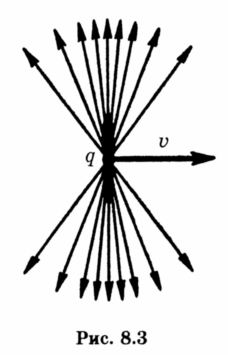«Thus, the consideration of the electric field of the simplest system - moving charge - shows that sometimes ГE ≠ 0, i.e., qualitatively new vortex electric field exists along with the potential.»
Fundamental physics course. A.D.Suhanov. 1998. Т.2. P.273. (In Russian)

If we analyse it more detailed, there are actually two vortex fields. One is related to the movement of the magnetic field, its electric induction lines are perpendicular to the motion direction and are not closed, it "flattens" the electric field. The other is related to the change (displacement) of an electric field in the space, it "tips" the charge field back. The vortex field is associated with electric displacement current, that flows in the opposite direction.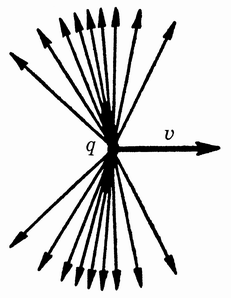«Example. The point charge q moves uniformly and linearly with a non-relativistic velocity v. Find the displacement current density vector at the point P, located at a distance r from the charge on a straight line perpendicular to its path and passing through the charge. Solution: jсм = -qv/r3.»
Electromagnetism. I.E.Irodov. 2000. С.302. (In Russian)
«In particular, the electric field at charge point moves not just moves with the charge, as in the case of infinitely high field propagation velocity, but changes in a more complicated manner. There are effects associated with the delayed emergence of the field at large distances from the charge ...»
Fundamentals of Physics. L.A.Gribov, N.I.Prokofev. 1995. P.300. (In Russian)

There is a figure in the encyclopedia, which shows the process of inclination of the field force lines.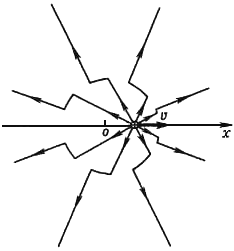«Fig. 1. The electric field force lines Е of the charge q, which began to move from a point o with the velocity u. ... The field, generated by moving charges, is propagated to the free from them space regardless of the sources with the same velocity c
Physical encyclopedia. ELECTRODYNAMICS. (In Russian)

Consequently in the result of the superposition of all fields at the charge motion the electric field not only "flattens", but also "lays" back. I hope, that the authors of textbooks on electrodynamics will take into account these notes and will provide the right figures. We should not forget, that the field does not propagate instantly when charge moves. It should be noted that, according to the Lorentz transformation law, the electric field of a moving charge only "flattens" and, according to electrodynamics, the field not only "flattens", but also "lays" back as a result of the delay electric field distribution while charge moves. This means, that from the point of view of electrodynamics, Lorentz transformations are idealistic, since they do not take into account the delayed spread of the induction field of the moving charge. It should also be taken into account, the vortex electric field emerge not only at the motion of the magnet, but at the charge motion, so the following statement is right: "the vortex electric field is generated by a variable magnetic field and a variable electric field both", but not like that, as it is written in the textbooks.

«The vortex electric field is generated by the variable magnetic field. Its force lines are always closed, like force lines of magnetic field.»
Physics. V.F.Dmitrieva. 2001. P.225. (In Russian)

Before introducing the fundamental postulate, which states that the vortex electric field force lines are always closed, it was necessary to consider all options of the magnetic field changes, including those, where the motion of the magnet is cross. That is, the consideration of the physical processes should not be one-sided. Faraday considered the longitudinal motion of the magnet, discovered electromagnetic induction, and the transverse motion of the magnet, which is of primary importance for the understanding of the electrodynamics of field processes, remains unconsidered. So, after Faraday no one tried just to turn the magnet cross and see what happens to the vortex fields. Thus, the longitudinal motion of the magnet generates vortex electric field with closed force lines and the transverse motion - vortex electric field, where the field lines are not closed, i.e. the induced electrical charges emerge.

«According to Maxwell, if every variable magnetic field excites in the surrounding space the vortex electric field, there should be the opposite statement: every change of the electric field should cause the emergence of the vortex magnetic field in the surrounding space.»
Physics course. T.I.Trofimova. 1998. P.248. (In Russian)

«Aristotle, in one of his works, wrote that fly has eight feet. But fly has only six feet. This error passed from a textbook to a textbook until 1872. The worship to the authority of the ancient teachers detains to see the obvious.»
http://rp-c.ru/index.php?id=209 (In Russian)

Physics is not based on faith, so any assertions should be verified, at that all possible options should be considered, but not just those, that do not contradict the postulate. One may ask: why it is necessary to deal with some kind of vortex fields? Without the understanding of the properties of the vortex fields, it is impossible to analyze the field structure of the photon, which like all electromagnetic waves, consist of vortex fields.

«... Gauss' theorem is valid not only for electrostatics, but also for electrodynamics, which using a variable in time electromagnetic fields. We are not sure if this hypothesis is valid or it is not valid ... Only the experiment can give the answer on this question. The whole collection of experimental facts speaks in favor of this hypothesis.»
General course of physics. Electricity. D.V.Sivuhin. 1996. V. 3. P. 1. P. 37. (In Russian)

In fact, Gauss theorem is true in electrostatics only. When it is extended to the variable fields, claiming that "the totality of the experience facts testifies this hypothesis," but it is not true, as such fact is forgotten to be reviewed, as the transverse motion of the magnet.

Now we shall sum up. The Solenoid electrical flux is always vortex. Nonsolenoid electrical flux can be either vortex or non-vortex. For example, in the transverse electromagnetic waves electric fluxes are vortex, but not solenoid, since all electrical induction lines are transverse to the direction of waves propagation and, accordingly, are not closed.

«... field in the transverse electromagnetic waves is purely vortex.»
Physical encyclopedia. THE ELECTRIC FIELD STRENGTH. (In Russian)
«... vectors E and H are perpendicular to the direction of the wave propagation ... vectors E and H in the electromagnetic wave is mutually orthogonal.»
Wave processes. I.E.Irodov. 1999. P.39. (In Russian)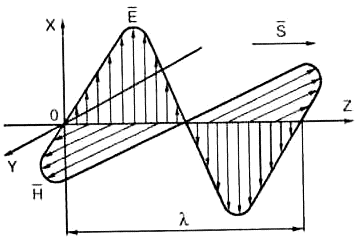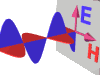So, in the textbooks in one place it is written that in the field in the electromagnetic waves is pure vortex, in the other, that the vortex field is always closed, in the third - that in the electromagnetic waves the vectors E and H are perpendicular to the direction of the wave propagation, but in no book on electrodynamics there is complete picture of such wave and induction lines are depicted open. It would be interesting to see how the induction lines of the vortex electric field perpendicular to the motion could be closed. This applies particularly to the rays and electromagnetic quanta. So that the electric induction could somehow become closed, the electric field strength should emerge in the longitudinal direction of waves propagation, which is not observed in the transverse electromagnetic waves - this is an experimental fact. The vortex electric field is closed only near the antenna, when the oscillations of the field have not yet turned into the propagating electromagnetic waves. The closure of magnetic induction lines in the electromagnetic waves is also under question.

The fact that the work of the vortex electric field at the motion of the electrical charge along a closed line can be different from zero, does not mean that the motion is on the induction line and does not speak, that the induction (power) lines are closed. It is said about the closed motion simply along the line, which generally may have various shapes, for example, the charge can move to one side and then the other. All field variables are vortex and when the charge in such field moves in one direction, one force acts on it, and when it comes back, because of the field power change, the other force acts on it, which induce the work of force.

The article "The Electrical Vortex Non-Solenoidal Fields" was published in the "New Energy Technologies" magazine, No. 3(6), 2002.

The complete text of the article is on
http://alemanow.narod.ru  (Russian / English)